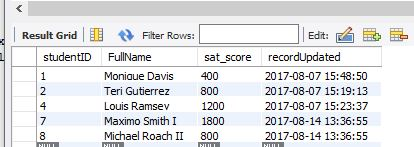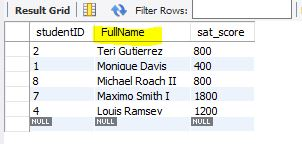# An introduction to SQL Syntax

An introduction to SQL Syntax
0

This guide provides a basic, high level description of the syntax for SQL statements.

SQL is an international standard (ISO), but you will find many differences between implementations. This guide uses MySQL as an example. If you use one of the many other Relational Database Managers (DBMS) you’ll need to check the manual for that DBMS if needed.

### What we will cover

• Use (sets what database the statement will use)
• Select and From clauses
• Where Clause (and / or, IN, Between, LIKE)
• Order By (ASC, DESC)
• Group by and Having

### How to use this

This is used to select the database containing the tables for your SQL statements:

``````use fcc_sql_guides_database; -- select the guide sample database
``````

### Select and From clauses

The Select part is normally used to determine which columns of the data you want to show in the results. There are also options you can use to show data that is not a table column.

This example shows two columns selected from the “student” table, and two calculated columns. The first of the calculated columns is a meaningless number, and the other is the system date.

``````	select studentID, FullName, 3+2 as five, now() as currentDate
from student;
``````

### Where Clause (and / or, IN, Between and LIKE)

The WHERE clause is used to limit the number of rows returned.

In this case all five of these will be used is a somewhat ridiculous Where clause.

Compare this result to the above SQL statement to follow this logic.

Rows will be presented that:

• Have Student IDs between 1 and 5 (inclusive)
• or studentID = 8
• or have “Maxmimo” in the name

The following example is similar, but it further specifies that if any of the students have certain SAT scores (1000, 1400), they will not be presented:

``````    select studentID, FullName, sat_score, recordUpdated
from student
where (
studentID between 1 and 5
or studentID = 8
or FullName like '%Maximo%'
)
and sat_score NOT in (1000, 1400);
``````### Order By (ASC, DESC)

Order By gives us a way to sort the result set by one or more of the items in the SELECT section. Here is the same list as above, but sorted by the students Full Name. The default sort order is ascending (ASC), but to sort in the opposite order (descending) you use DESC, as in the example below:

``````    select studentID, FullName, sat_score
from student
where (studentID between 1 and 5 -- inclusive
or studentID = 8
or FullName like '%Maximo%')
and sat_score NOT in (1000, 1400)
order by FullName DESC;
``````### Group By and Having

Group By gives us a way to combine rows and aggregate data. The Having clause is like the above Where clause, except that it acts on the grouped data.

This data is from the campaign contributions data we’ve been using in some of these guides.

This SQL statement answers the question: “which candidates recieved the largest number of contributions (not \$ amount, but count (*)) in 2016, but only those who had more than 80 contributions?”

Ordering this data set in a descending (DESC) order places the candidates with the largest number of contributions at the top of the list.

``````    select Candidate, Election_year, sum(Total_\$), count(*)
from combined_party_data
where Election_year = 2016
group by Candidate, Election_year
having count(*) > 80
order by count(*) DESC;
``````As with all of these SQL things there is MUCH MORE to them than what’s in this introductory guide. I hope this at least gives you enough to get started. Please see the manual for your database manager and have fun trying different options yourself.